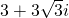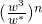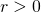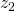# Random Questions from 2016 Prelims #11TPJC/P2/4

(a) The complex number w is given by(i) Find the modulus and argument of w, giving your answer in exact form.
(ii) Without using a calculator, find the smallest positive integer value of n for whichis a real number.

(b) The complex number z is such that(i) Find the value of z in the form, whereand.
(ii) Show the roots on an argand diagram.
(iii) The roots represented byandare such that. The locus of all points z such thatintersects the line segment joining points representingandat the point P. P represents the complex number p. Find, in exact form, the modulus and argument of p.

Not readable? Change text.